# The two-sum problem in JavaScriptself.__wrap_n=self.__wrap_n||(self.CSS&&CSS.supports("text-wrap","balance")?1:2);self.__wrap_b=(e,r,t)=>{t=t||document.querySelector(`[data-br="\${e}"]`);let n=t.parentElement,a=s=>t.style.maxWidth=s+"px";t.style.maxWidth="";let o,i=n.clientWidth,l=n.clientHeight,c=i/2-.25,f=i+.5;if(i){for(a(c),c=Math.max(t.scrollWidth,c);c+1<f;)o=Math.round((c+f)/2),a(o),n.clientHeight===l?f=o:c=o;a(f*r+i*(1-r))}t.__wrap_o||typeof ResizeObserver<"u"&&(t.__wrap_o=new ResizeObserver(()=>{self.__wrap_b(0,+t.dataset.brr,t)})).observe(n)};self.__wrap_n!=1&&self.__wrap_b(":Rj0pm:",1)

October 30, 2023

The two-sum problem is a common interview challenge that asks for a function to find the indices of the two numbers in a given array that add up to a specific target. Engineers appreciate solutions that are both efficient and easy to understand.

## Initial approach with a brute force solution

A brute force approach involves checking every possible pair in the array to see if they add up to the target sum. This is not the most efficient solution but is straightforward to understand.

```function twoSumBruteForce(nums, target) {
for (let i = 0; i < nums.length; i++) {
for (let j = i + 1; j < nums.length; j++) {
if (nums[i] + nums[j] === target) {
return [i, j];
}
}
}
}```

## Optimizing with a hash map

To optimize, you can use a JavaScript object as a hash map, storing the difference between the target and the current element as keys, and their indices as values. This allows for constant-time lookups.

```function twoSumHashMap(nums, target) {
let numIndices = {};
for (let i = 0; i < nums.length; i++) {
let complement = target - nums[i];
if (numIndices[complement] !== undefined) {
return [numIndices[complement], i];
}
numIndices[nums[i]] = i;
}
}```

## Edge cases to consider

While implementing the two-sum function, edge cases such as duplicate numbers that add up to the target or invalid input should be considered.

```function twoSumHandleEdges(nums, target) {
let numIndices = {};
for (let i = 0; i < nums.length; i++) {
if (numIndices.hasOwnProperty(target - nums[i])) {
return [numIndices[target - nums[i]], i];
}
numIndices[nums[i]] = i;
}
throw new Error('No two sum solution');
}```

Thoroughly test the two-sum function to ensure it handles various scenarios correctly. Use a diverse set of test cases including positive and negative numbers, and edge cases.

```console.log(twoSumHashMap([2, 7, 11, 15], 9)); // [0, 1]
console.log(twoSumHashMap([3, 2, 4], 6)); // [1, 2]
console.log(twoSumHashMap([3, 3], 6)); // [0, 1]
try {
console.log(twoSumHandleEdges([1, 2, 3], 7)); // Error
} catch (e) {
console.error(e.message);
}```

## Considerations for large datasets

For very large datasets, it’s crucial to consider the time complexity. The brute force method is O(n^2), while the hash map approach brings it down to O(n). Always prefer algorithms that scale well with large input sizes.

## ES6+ improvements

With the advent of new JavaScript syntax, the solution can be more concise using ES6 features like the `Map` object for better performance and clearer intent.

```function twoSumES6(nums, target) {
const numMap = new Map();
for (let i = 0; i < nums.length; i++) {
const complement = target - nums[i];
if (numMap.has(complement)) {
return [numMap.get(complement), i];
}
numMap.set(nums[i], i);
}
}```

## Time and Space Complexity Analysis

It's important to analyze both the time and space complexity of each solution:

• The brute force approach has a time complexity of O(n^2) because it uses two nested loops, and a space complexity of O(1) since it doesn't allocate additional space proportional to the input size.
• The hash map approach has a time complexity of O(n), since it processes each element once, and a space complexity of O(n) as well, due to the additional storage used by the hash map.

## Testing and Validation

Create comprehensive test suites using frameworks like Jest to cover all possible scenarios, including but not limited to:

• Arrays with negative numbers
• Arrays with zeros and repeated numbers
• Non-integer numbers
• Empty arrays or arrays with one element

## Handling Large Numbers

In JavaScript, numerical precision issues can occur with very large numbers. Consider using libraries like `BigInt` for arbitrary precision arithmetic if you expect to handle large integers.

# Ship faster, worry less with Basedash

You're busy enough with product work to be weighed down building, maintaining, scoping and developing internal apps and admin panels. Forget all of that, and give your team the admin panel that you don't have to build. Launch in less time than it takes to run a standup.

You're busy enough with product work to be weighed down building, maintaining, scoping and developing internal apps and admin panels. Forget all of that, and give your team the admin panel that you don't have to build. Launch in less time than it takes to run a standup.

You're busy enough with product work to be weighed down building, maintaining, scoping and developing internal apps and admin panels. Forget all of that, and give your team the admin panel that you don't have to build. Launch in less time than it takes to run a standup.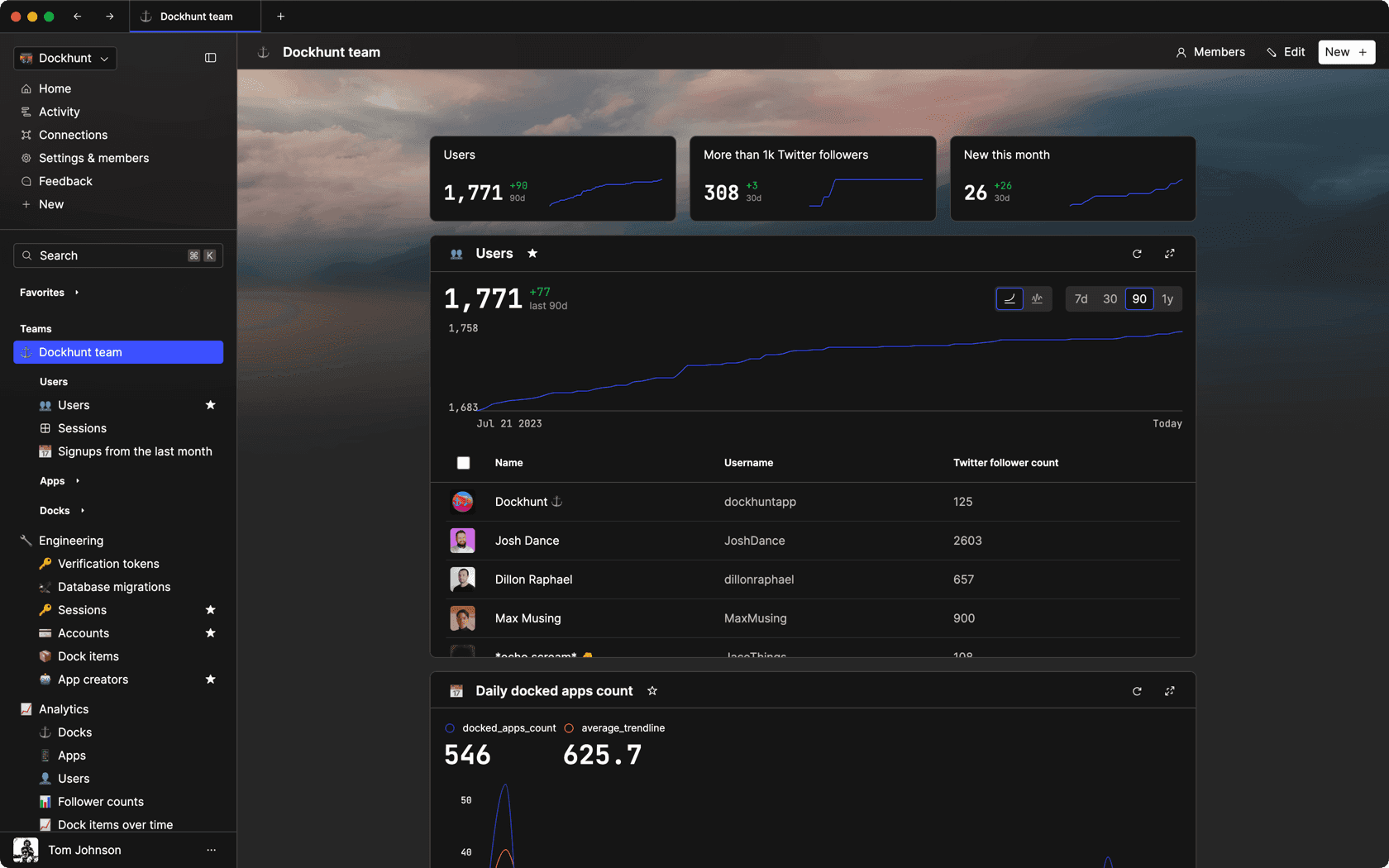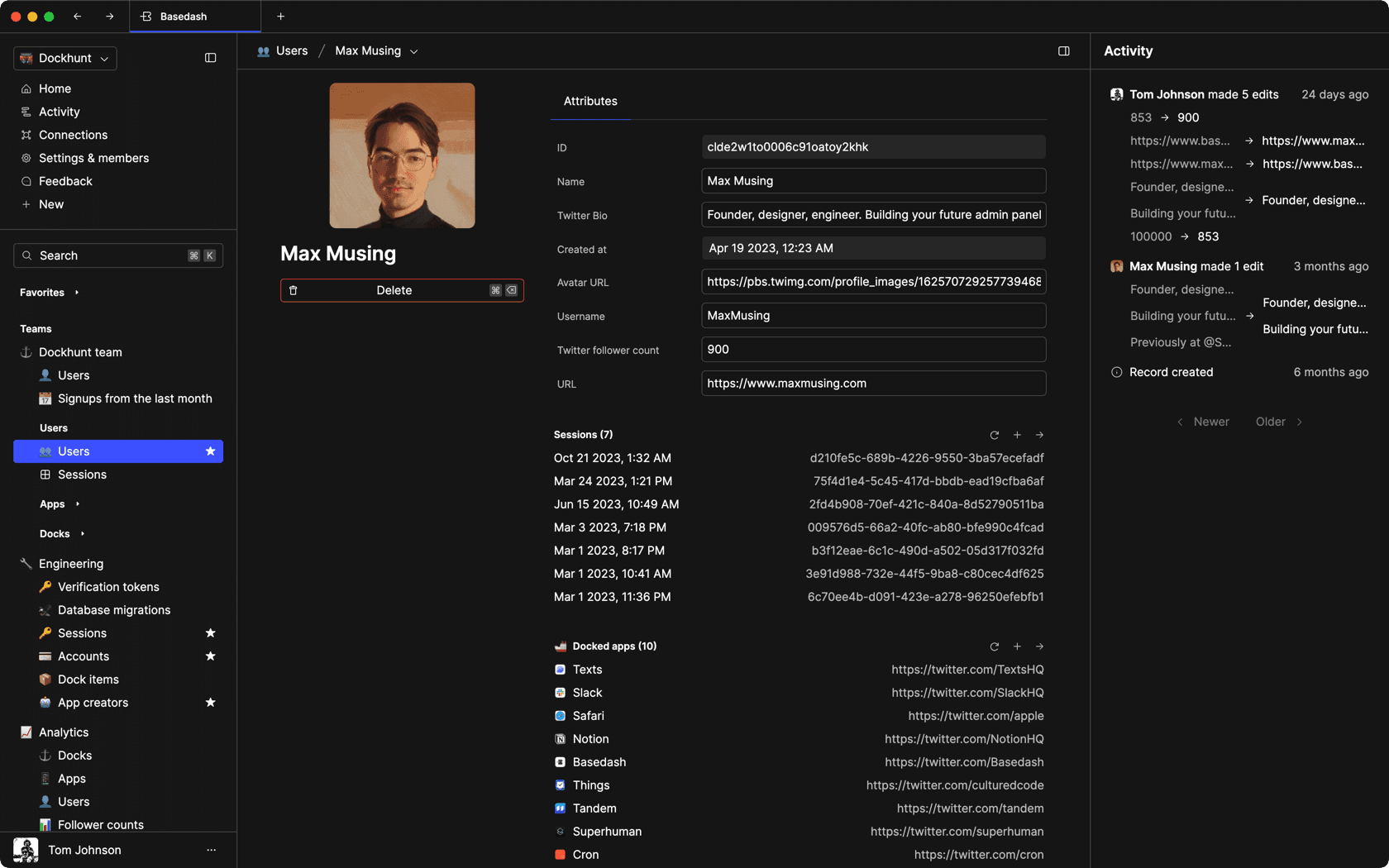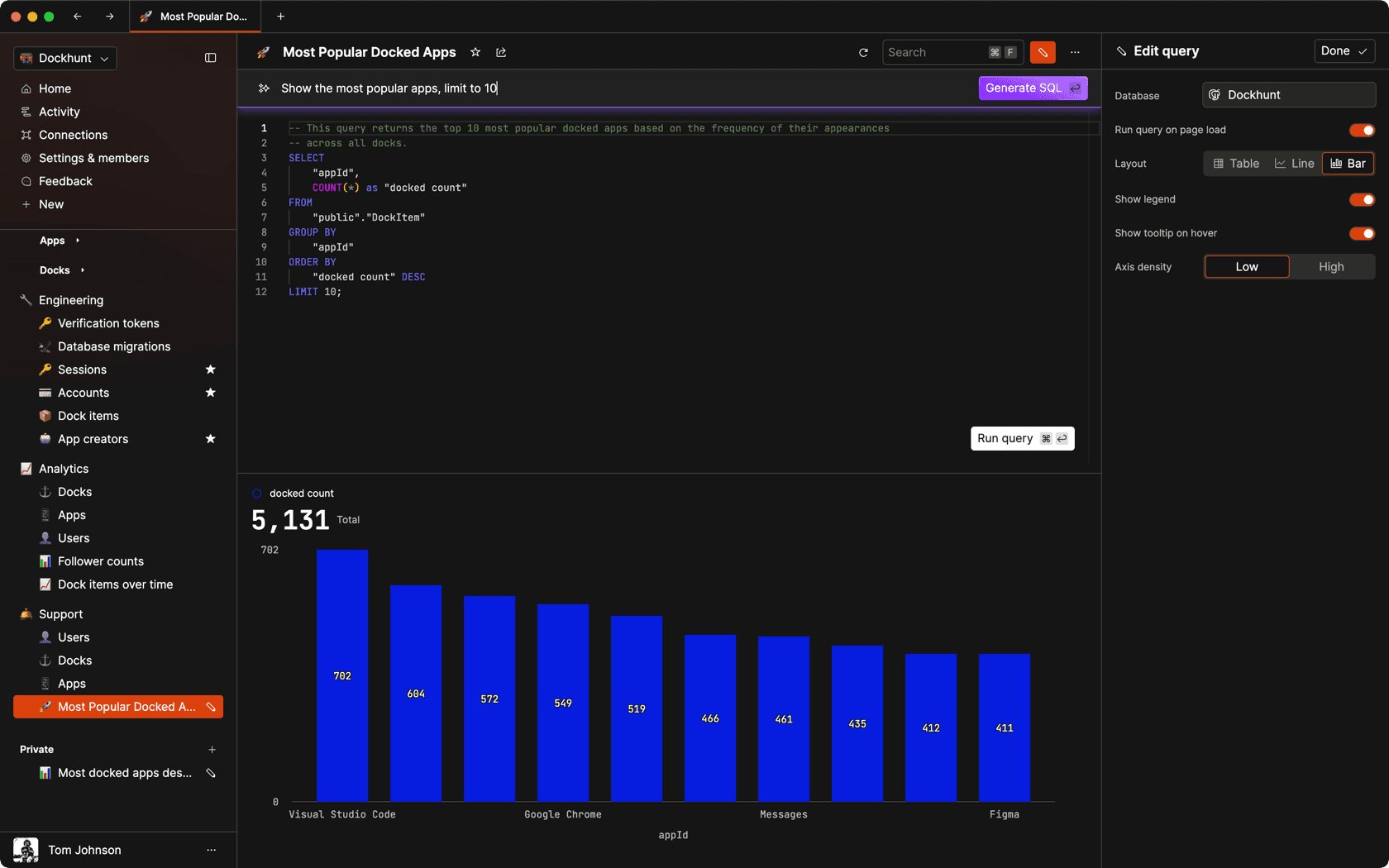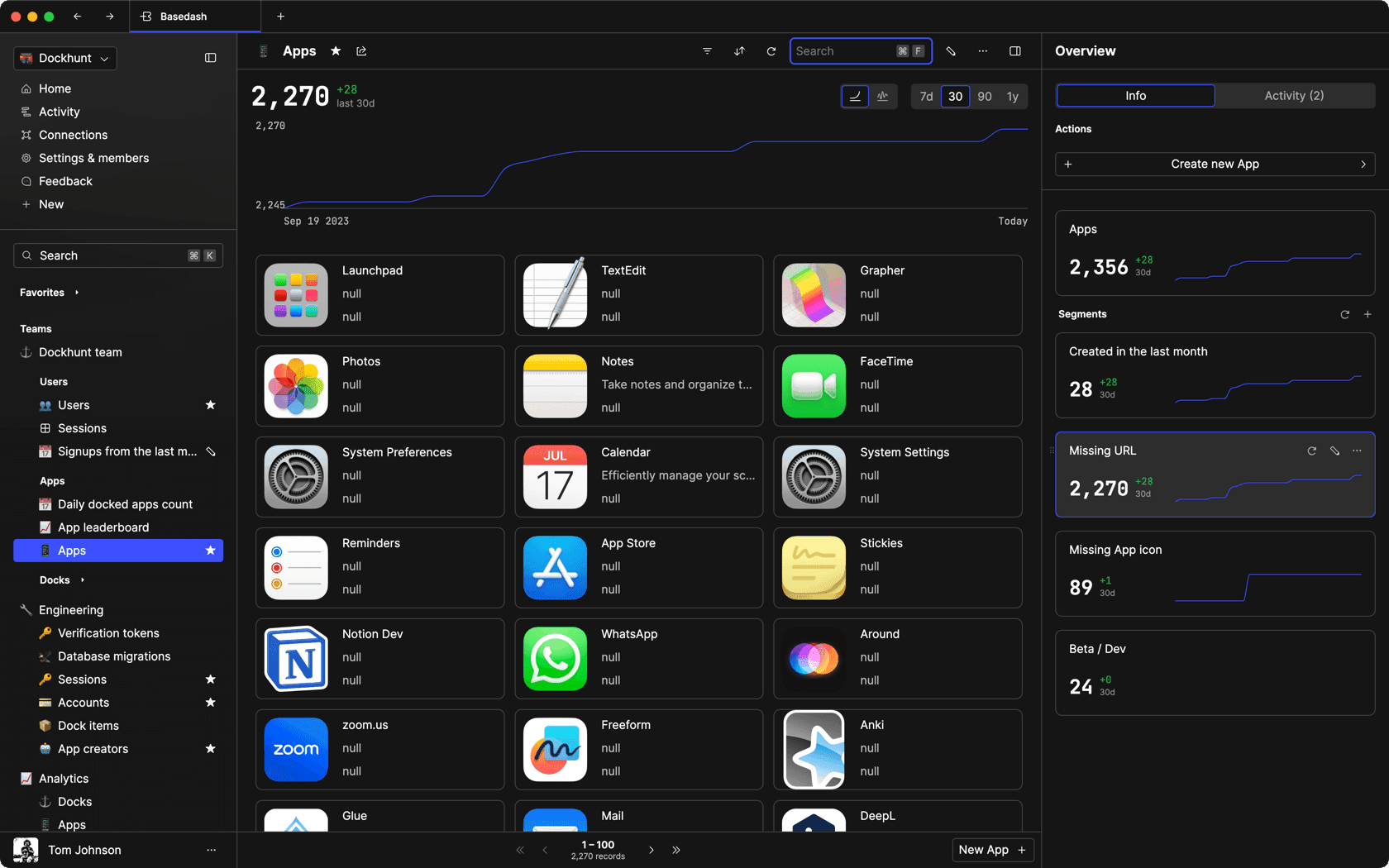### Dashboards and charts

Effortlessly make charts and create a space for your team to work together towards shared goals and metrics.

### SQL composer with AI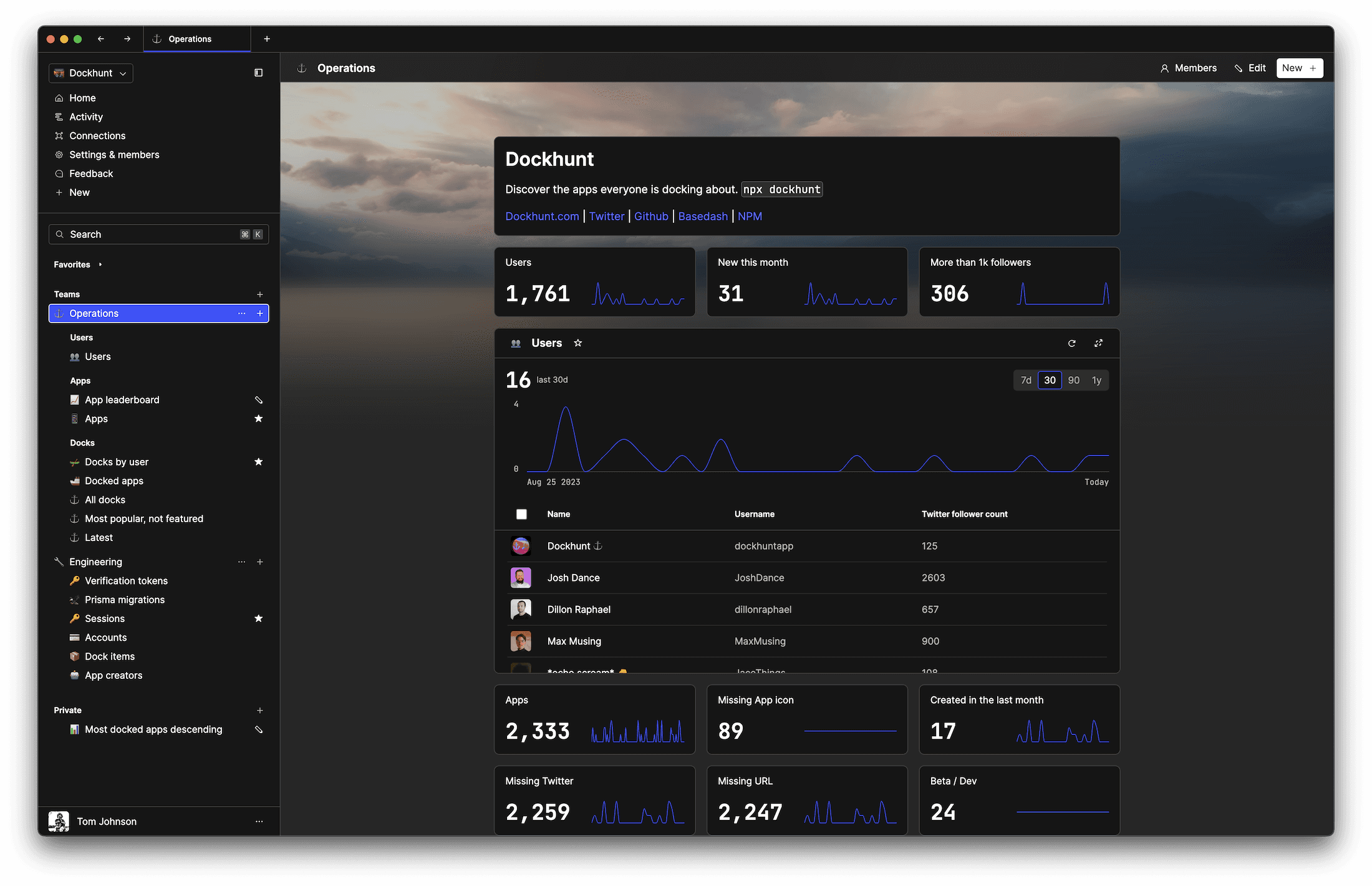SOC II
Type 2 Certified

### Features

Proudly carbon-neutral.SOC II
Type 2 Certified

### Features

Proudly carbon-neutral.SOC II
Type 2 Certified